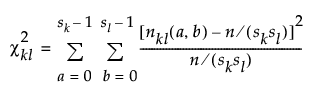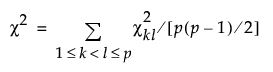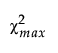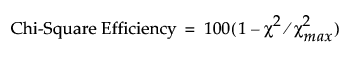Publication date: 11/10/2021

## Main Effects Screening Designs

If an experiment involves categorical or discrete numeric factors, or if the number of runs is constrained, it might not be possible to construct an orthogonal design for screening main effects. However, a main effects screening design can be constructed. See Lekivetz et al. (2015).

A main effects screening design is a design with good balance properties as described by a Chi-square criterion. See Chi-Square Efficiency. Such designs have desirable statistical properties for main effect models.

The algorithm used to generate the design attempts to construct an orthogonal array of strength two. Strength-two orthogonal arrays permit orthogonal estimation of main effects when interactions are negligible. These arrays are ideal for screening designs. Regular fractional factorial designs of Resolution 3 and Plackett-Burman designs are examples of strength-two orthogonal arrays.

Consider all possible pairs of levels for factors in the design. The algorithm attempts to balance the number of pairs of levels as far as possible. Given that a fixed number of columns has been generated, a new balanced column is randomly constructed. A measure is defined that reflects the degree of balance achieved for pairs that involve the new column. The algorithm attempts to minimize this measure by interchanging levels within the new column.

### Chi-Square Efficiency

Suppose that a design has n runs and p factors corresponding to the columns of the design matrix.

Denote the levels of factors k and l by a = 0, 1, , sk 1and b = 0, 1, , sl 1, respectively.

Denote the number of times that the combination of levels (a,b) appears in columns k and l by nkl(a,b).

A measure of the lack of orthogonality evidenced by columns k and l is given by the following expression:A measure of the average non-orthogonality of the design is given by this expression:The maximum possible value of χ2, denoted, is obtained. The chi-square efficiency of a design is defined as follows:Chi-square efficiency indicates how close χ2 is to zero, relative to a design in which pairs of levels show extreme lack of balance.